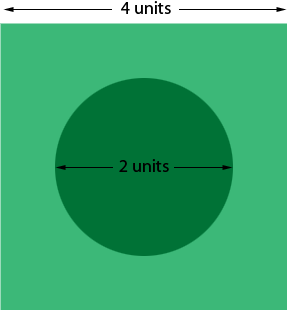SEARCH HOMEMath Central Quandaries & QueriesQuestion from Jenn, a student: A circle of a diameter of 2 is drawn inside a square of 4. The circle's center is at the center of the square. To the nearest tenth, what is the perimeter of the area of the square, not including the circle inside.Hi Jenn,

I think the question should say

A circle of a diameter of 2 is drawn inside a square of side length 4. The circle's center is at the center of the square. To the nearest tenth, what is the area of the region of the square not inside the circle.

Here is my diagram.The square has side length 4 units. What is its area?

The circle has radius 1 unit. What is its area?

What is the difference in the areas?

I may be interpreting the problem incorrectly. If so, let me know.

PennyMath Central is supported by the University of Regina and The Pacific Institute for the Mathematical Sciences.